# Dynamique de la Pyramide des Ages

Très joli billet sur blog.revolutionanalytics.com avec un code de @kyle_e_walker permettant, très simplement (moyennant une inscription pour avoir une clé permettant d’utiliser l’API du census) de construire une pyramide des âges dynamiques.

```> devtools::install_github('walkerke/idbr')
> library(idbr)
> library(ggplot2)
> library(animation)
> library(dplyr)
> library(ggthemes)
> idb_api_key("mykey1239F2f324zf9GGZgege32R2ii4")```

On importe alors les données pour les hommes et les femmes,

```> male <- idb1('FR', 2010:2050, sex = 'male') %>%
+   mutate(POP = POP * -1,
+   SEX = 'Male')
> female <- idb1('FR', 2010:2050, sex = 'female') %>% mutate(SEX = 'Female')```

et on stocke le tout

```> france <- rbind(male, female) %>%
+   mutate(abs_pop = abs(POP))```

Ensuite, on crée l’animation,

```> saveGIF({
+
+   for (i in 2010:2050) {
+
+     title <- as.character(i)
+
+     year_data <- filter(france, time == i)
+
+     g1 <- ggplot(year_data, aes(x = AGE, y = POP, fill = SEX, width = 1)) +
+       coord_fixed() +
+       coord_flip() +
+       annotate('text', x = 98, y = -800000,
+       label = 'Data: US Census Bureau IDB; idbr R package', size = 3) +
+       geom_bar(data = subset(year_data, SEX == "Female"), stat = "identity") +
+       geom_bar(data = subset(year_data, SEX == "Male"), stat = "identity") +
+       scale_y_continuous(breaks = seq(-1000000, 1000000, 500000),
+       labels = paste0(as.character(c(seq(1, 0, -0.5), c(0.5, 1))), "m"),
+       limits = c(min(france\$POP), max(france\$POP))) +
+       theme_economist(base_size = 14) +
+       scale_fill_manual(values = c('#ff9896', '#d62728')) +
+       ggtitle(paste0('Population structure of France, ', title)) +
+       ylab('Population') +
+       xlab('Age') +
+       theme(legend.position = "bottom", legend.title = element_blank()) +
+       guides(fill = guide_legend(reverse = TRUE))
+     print(g1)
+   }
+ }, movie.name = 'france_pyramid.gif', interval = 0.1, ani.width = 700, ani.height = 600)```Et le résultat est vraiment joli, non ?

# How old is the oldest person you know?

Last week, we had a discussion with some colleagues about the fact that – in order to prepare for the SOA exams – we did not have time (so far) to mention results on extreme values in our actuarial program. I did gave an introduction in my nonlife actuarial models class, but it was only an introduction, in three hours, in order to illustrate reinsurance pricing. And I told my students that if they wanted to know more about extreme values, they should start a master program in actuarial science and finance, since I will give a course on extremes (and copulas) next winter.

But actually, extreme values are everywhere ! For instance, there is a Prudential TV commercial where has people place large, round stickers on a number line to represent the age of the oldest person they know. This forms some kind of histogram. The message is to have Prudential prepare you to have adequate money for all these years. And actually, anyone can add his or her own sticker at the Prudential website.Patrick Honner, on his blog (http://mrhonner.com/…), did mention this interesting representation. But this idea is not new, as mentioned in a post, published three years ago. In 1932, Emil Gumbel gave a talk in France on the “âge limite“. And as he wrote it “on peut donc supposer que la distribution de l’âge limite – c’est à dire la probabilité que cet âge ait une valeur donnée – soit Gaussienne“. In 1932 (not aware of Fisher and Tippett work, he thought that the limiting distribution for a maximum would be Gaussian). But a few years after, he read about Fisher’s work, and observed also that “la distribution d’une valeur extrêmes peut être représentée pour un nombre suffisant d’observations par la formule doublement exponentielle, pourvu que la distribution initiale se comporte asymptotiquement comme une exponentielle. La formule devient rigoureuse si la distribution initiale est exponentielle“, as he wrote in 1935. And in 1937, he wrote a paper on “les centennaires” that can also be related to the work of Bortkiewicz on rare events. One should also mention one of the most important paper in extreme value theory, published in 1974 by Balkema and de Haan, on Residual Life Time at Great Age.

Because in this experiment, the question is “How Old is the Oldest Person You Know?“, so it is the distribution of a maximum. And from Fisher-Tippett theorem, if we assume that the age is bounded (and that there exists some finite upper limit), then the limiting distribution for the maxima (or to be more rigorous, a affine transformation of the maxima) should be Weibull distribution. And this is what it looks like

`> plot(-x,dweibull(x,2.25,4),type="l",lwd=2)`As an actuary, the only thing I know about demography, is the distribution of the age of death. For instance, consider the following French life table

```> alive <- read.table(
+ "https://perso.univ-rennes1.fr/arthur.charpentier/TV8890.csv",
> nb= -diff(alive)
> ages=0:110
> plot(ages,nb,type="h")```This is the distribution of the age of the death in a given population. Which is not the same as the distribution mentioned above! What we look for is the following: given that someone is alive, what could be the distribution of his-her age ? Actually, if we assume that the yearly number of birth is constant with time (as well as death probability), then we can compute easily to number of people of age  : we take everyone born (exactly) years ago, and remove all those who died at at , , etc. So the function should be

```> probadeath=nb/sum(nb)
> surv=Vectorize(nbx)(ages)
> distrage=surv/sum(surv)```

which looks likeBut this assumption of constant number of birth is not that relevent. And actually, what we need is the distribution of the age within a population… This is a population pyramid, actually. The French one can be downloaded from http://www.insee.fr/fr/ppp/bases-de-donnees/….

```> population <- read.table("popinsee2007.csv",sep=";",header=TRUE)\$POPTOT07
> ages=0:107
> plot(ages,population/sum(population),type="h")```(the red line being the one obtained previously, using some natality assumptions). Now, let us use this population to generate acquaintances.

```> agemax=function(nsim=1000,size=20){
+ agemax=rep(NA,nsim)
+ for(i in 1:nsim){
+ X=sample(ages,prob=population/sum(population),size=size,replace=TRUE)
+ agemax[i]=max(X)}
+ return(agemax)}```

Here, we assume that everyone knows 20 other people, randomly chosen in the entire population, then we return the age of the oldest. And we do that for 1,000 people. Here is the distribution, we obtain

```> XS=agemax(10000,20)
> plot(table(XS)/length(XS),type="h",xlim=c(0,108))```where the red line is a Weibull distribution (a transformed one, actually, since in extremely value theory, the distance to the upper bound of the distribution has a Weibull density),

```> library(MASS)
> fit=fitdistr(108-XS,dweibull,list(shape=1,scale=1))
> lines(ages,dweibull(108-ages,fit\$estimate,fit\$estimate),col="red")```

Which is quite close to the distribution obtained in the commercial, don’t you think ? But still, it should be possible to be more accurate, since people should think of their parents, or grandparents. So I guess it could be possible to build a more accurate algorithm, to get something closer to the distribution obtained on the Prudential website. But first, let us wait to have more stickers, more observations… and then I’ll be back to play with it !

# Les députés sont-ils à l’image de la population

Beaucoup de choses ont été écrites sur le fait que les députés ne sont pas vraiment le reflet de la population, que ce soit en terme de profession, de sexe, d’origine, d’age, etc. La liste pourrait être longue. Il y a plusieurs mois, j’avais commencé à regarder le profil des députés, par age. En effet, le site http://assemblee-nationale.fr/ permet d’accéder à des données sur tous les députés, depuis la Révolution. Y compris leur date de naissance. En croisant ces données avec des données de population, par exemple via http://www.mortality.org/, on peut comparer la répartition des ages des députés, avec la répartition des ages de la population.

Pour les amateurs, le code pour récupérer les données (ou au moins les dates de naissance des députés) ressemble à

```N=2002
URL=paste("http://www.assemblee-nationale.fr/
regle_nom=est&Nom=&departement=&
choixdate=intervalle&D%C3%A9butMin=01%2F01%2F",
N,"&FinMin=31%2F12%2F",N,"&Dateau=&legislature=",
s,"&choixordre=chrono&Rechercher=
Lancer+la+recherche",sep="")
HTML=scan(URL,what="character")

k=which(HTML=="class=\"titre\">Né")
vHTML=HTML[k:length(HTML)]
vk=which(substr(vHTML,1,7)==">&nbsp;")
liste=vHTML[vk]
naissance=liste[seq(1,length(liste),by=2)]
NAISSANCE=as.Date(substr(naissance,8,17),
"%d/%m/%Y")```

Maintenant, pour être tout à fait honnête, je ne suis pas certain de ce qui est vraiment renvoyé, et j’ai des doutes que cela correspondent réellement à la requête faite. En effet, même si je demande à avoir la liste des députés après l’élection, j’ai trop de monde… mais peut-être est-ce du aux décès éventuels, et il est possible que l’ensemble des députés qui ont siégé pendant la mandature apparaissent dans le résultat de la requête.

Sur la figure suivante on voit, sur plusieurs élections depuis plus de 100 ans, comment les deux distributions se déforment, avec en rouge la distribution de l’age des députés, et en bleu, la distribution de la population française, dans son ensemble (population de plus de 18 ans)Si on veut tout suivre sur un graphique, au lieu de se regarder une animation, on peut représenter les différents quantiles (10%, 25%, 75% et 90%, retenus sur la population de plus de 18 ans, et l’age médian, au centre), avec la population française l’année de l’élection,et l’ensemble des élus au parlement,Si on veut faciliter la comparaison, on peut se contenter de visualiser l’évolution des ages moyens,ou encore, du ratio (en % de différence) entre l’age moyen des députés, et celui de l’ensemble de la population.Sur ce graphique, on voit que depuis 30 ans, l’age moyen des députés croit plus vite que celui de la population: la population français vieilli, mais moins que ses députés… La gérontocratie perdure donc en France. En espérant que cela ne débouche pas sur le clash générationnel que l’on semble observer ces temps-ci au Québec…

# Infidelity and econometricsOn http://www.bakadesuyo.com, there was recently an interesting discussion about infidelity, the key question being “at what ages are men and women most likely to have affairs?” The discussion is based on some graphs, e.g.The source is a paper by Donald Cox. Based on a sample of 36 men and 22 women 3,432 respondent (NHSLS dataset) . And to be honest, I have been surprised by the shape of the curves. Especially for men… In order to compare, it is possible to use another dataset that can be found in R,

```> library(Ecdat)
> data(Fair)
> tail(Fair)
sex age   ym child religious education occupation
596   male  47 15.0   yes         3        16          4
597   male  22  1.5   yes         1        12          2
598 female  32 10.0   yes         2        18          5
599   male  32 10.0   yes         2        17          6
600   male  22  7.0   yes         3        18          6
601 female  32 15.0   yes         3        14          1
rate nbaffairs
596    2         7
597    5         1
598    4         7
599    5         2
600    2         2
601    5         1```

with 601 observations (from Fair (1977)). It is possible to run a Poisson regression to describe the number of affairs in the past year. E.g for men

```> library(splines)
> regM=glm(nbaffairs~bs(age),family=poisson,
+ data=Fair[Fair\$sex=="male",])
> a=seq(20,60)
> N=predict(regM,newdata=data.frame(age=a),type="response")
> plot(a,N,type="l",lwd=2,col="red")```or for women,

```> regF=glm(nbaffairs~bs(age),family=poisson,
+ data=Fair[Fair\$sex=="female",])
> N=predict(regF,newdata=data.frame(age=a),type="response")
> plot(a,N,type="l",lwd=2,col="red",lty=2)```On that (larger) dataset, we obtain curves that are more intuitive… But maybe the Poisson distribution is not an appropriate model. For instance, having no affairs do not mean that the person did not want to… So perhaps, a more interesting model would be a Poisson model with a zero-inflation, i.e. some people are honest and do not want to have affairs (and appear as 0), while some do want to have some affairs, and the number of affairs is Poisson distributed (and can take the value 0). If we focus on people wo do not want to have affairs, the model (and the prediction) is the following, where we plot the probability of not being interested in having an affair,

```> library(pscl)
> regM0=zeroinfl(nbaffairs~bs(age)|bs(age),family=poisson,
> N0=predict(regM0,newdata=data.frame(age=a),type="zero")
> plot(a,N0,type="l",lwd=2,col="blue")```For those willing to have an affair, here is the parameter of the Poisson distribution of the number of affairs,

```> Nc=predict(regM0,newdata=data.frame(age=a),type="count")
> plot(a,Nc,type="l",lwd=2,col="purple")```The same can be done for women, with the probability of no-willing to have an affair,and to Poisson rate for women willing to have an affair,If we focus on people willing to have an affair, the curves are the following,i.e. men below 40 have more interested, but after 40, the probability drops, while women are still more and more likely to be willing to have an affair. On the other hand, young women having affairs might be less, but they usually have much more affairs than men…# Oscar awards: good actor versus good actressI am not a big fan of those ceremonies, where some actors pretend that they are extremely happy to be there, and then some win a trophy, some don’t, and those who win start to cry, and those who did not get a trophy try to pretend that they are not affected, etc. The other reason is that, since I have several kids, I do not go to see the movies that often (I mean apart from Shrek, Toy Story… Harry Potter is probably the only movie I’ve seen with real actors – or at least human actors).

But I remember being surprised when I looked at the nominees in newspapers,Actresses are beautiful and look young, while actors are more experienced. So I have try to see how old were those who win an Oscar, as best actor (here) or best supporting actor (there), and best actress (here) and best supporting actress (there).

```OSCAR=read.table("http://freakonometrics.blog.free.fr/public/data/OSCAR.csv",
actor=OSCAR[,1]
suppactor=OSCAR[,2]
actress=OSCAR[,3]
suppactress=OSCAR[,4]
actor=actor[is.na(actor)==FALSE]
actor=actor[actor>0]
actress=actress[is.na(actress)==FALSE]
actress=actress[actress>0]
suppactor=suppactor[is.na(suppactor)==FALSE]
suppactor=suppactor[suppactor>0]
suppactress=suppactress[is.na(suppactress)==FALSE]
suppactress=suppactress[suppactress>0]

boxplot(actor,suppactor,actress,suppactress,col=c("blue","blue","red","red"),
names=c("actor","supp. actor","actress","supp. actress"))```On average, a best actress is 36 years old, while a best actor is 44 years old.  Which is quite a difference… Perhaps because it takes more time to an actor to be a good one ? Assuming that they start acting at 18, it takes 18 more years for an actress to be recognized as a good one (here the best one), and 26 for an actor. Or perhaps it is simply because leading actresses have to look young…
The oldest actor who won an Oscar was Henry Fonda (at the age of 76) and the oldest actress was Jessica Tendy (nearing 81). Tatum O’Neal became the youngest person to win the best suppo
rting actress award
at the age of 10 (she was 8 when she was acting). The youngest best actress was Marlee Matlin, 21. The distribution was be seen below, with actors in blue, and actresses in red, best supporting actors in dotted lines, and best actors in plain lines,

```plot(density(actor),xlim=c(10,80),axes=FALSE,
col="blue",names="",ylab="",xlab="",ylim=c(0,.051))
lines(density(suppactor),col="blue",lty=2)
lines(density(actress),col="red")
lines(density(suppactress),col="red",lty=2)
axis(1)```Note that the age of supporting actors is older that leading ones. E.g. the average age for supporting actors winning an Oscar is 50, while it is  44 for actors. Similarly, it is 40 for supporting actresses, and 36 for actresses.

```> mean(suppactor)
 50.23762

> mean(actor)
 44.29982

> mean(suppactress)
 40.55766

> mean(actress)
 36.39733```

Here, I have to admit that I was surprised. I always thought that being a supporting actor was a first step before being a leading one. So winners of supporting awards should have been younger that winners of leading ones. But this is not the case.

And the dynamic here is rather stable, with actors,and actresses,except that the age difference between supporting roles and leading roles have increased in the 80’s for actors, while it decreased in the 80’s for actresses.

# Open data might be a false good opportunity…

I am always surprised to see many people on Twitter tweeting about #opendata, e.g. @data4all, @usdatagov, @datapublicatwit, @ProPublica or @open3 among so many others… Initially, I was also very enthousiastic, but I have to admit thatopen data are rarely raw data. Which is what I am usually looking for, as a statistician…
Consider the following example: I was wondering (Valentine’s day is approaching)when will a man born in 1975 (say) get married – if he ever gets married ?More technically, I was looking for a distribution of the age of first marriage (given the year of birth), including the proportion of men that will never get married, for that specific cohort.The only data I found on the internet is the following, on statistics.gov.uk/Note that we can also focus on women (e.g. here). Is it possible to use that opendata to get an estimation of the distribution of first marriage for some specific cohort ? (and to answer the question I asked). Here, we have two dimensions: on line, the year (of the marriage), and on column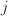, the age of the man when he gets married. Assume that those were rawdata, i.e. that we have the number of marriages of men of ageduring the year.

We are interested at a longitudinal lecture of the table, i.e. consider some man born year, we want to estimate (or predict) the age he will get married, if he gets married. With raw data, we can do it… The first step is to build up triangles (to have a cohort vs. age lecture of the data), and then to consider a model, e.g.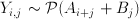where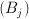is a year effect, and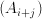is a cohort effect.

```base=read.table("http://freakonometrics.free.fr/mariage-age-uk.csv",
m=base[1:16,]
m=m[,3:10]
m=as.matrix(m)
triangle=matrix(NA,nrow(m),ncol(m))
n=ncol(m)
for(i in 1:16){
triangle[i,]=diag(m[i-1+(1:n),])
}
triangle[nrow(m),1]=m[nrow(m),1]

triangle
[,1] [,2] [,3] [,4] [,5] [,6] [,7] [,8]
[1,]   12  104  222  247  198  132   51   34
[2,]    8   89  228  257  202  102   75   49
[3,]    4   80  209  247  168  129   92   50
[4,]    4   73  196  236  181  140   88   45
[5,]    3   78  242  206  161  114   68   47
[6,]   11  150  223  199  157  105   73   39
[7,]   12  117  194  183  136   96   61   36
[8,]   11  118  202  175  122   92   62   40
[9,]   15  147  218  162  127   98   72   48
[10,]   20  185  204  171  138  112   82   NA
[11,]   31  197  240  209  172  138   NA   NA
[12,]   34  196  233  202  169   NA   NA   NA
[13,]   35  166  210  199   NA   NA   NA   NA
[14,]   26  139  210   NA   NA   NA   NA   NA
[15,]   18  104   NA   NA   NA   NA   NA   NA
[16,]   10   NA   NA   NA   NA   NA   NA   NA

Y=as.vector(triangle)
YEARS=seq(1918,1993,by=5)
AGES=seq(22,57,by=5)
X1=rep(YEARS,length(AGES))
X2=rep(AGES,each=length(YEARS))
reg=glm(Y~as.factor(X1)+as.factor(X2),family="poisson")
summary(reg)

Call:
glm(formula = Y ~ as.factor(X1) + as.factor(X2), family = "poisson")

Deviance Residuals:
Min       1Q   Median       3Q      Max
-5.4502  -1.1611  -0.0603   1.0471   4.6214

Coefficients:
Estimate Std. Error z value Pr(>|z|)
(Intercept)        2.8300461  0.0712160  39.739  < 2e-16 ***
as.factor(X1)1923  0.0099503  0.0446105   0.223 0.823497
as.factor(X1)1928 -0.0212236  0.0449605  -0.472 0.636891
as.factor(X1)1933 -0.0377019  0.0451489  -0.835 0.403686
as.factor(X1)1938 -0.0844692  0.0456962  -1.848 0.064531 .
as.factor(X1)1943 -0.0439519  0.0452209  -0.972 0.331082
as.factor(X1)1948 -0.1803236  0.0468786  -3.847 0.000120 ***
as.factor(X1)1953 -0.1960149  0.0470802  -4.163 3.14e-05 ***
as.factor(X1)1958 -0.1199103  0.0461237  -2.600 0.009329 **
as.factor(X1)1963 -0.0446620  0.0458508  -0.974 0.330020
as.factor(X1)1968  0.1192561  0.0450437   2.648 0.008107 **
as.factor(X1)1973  0.0985671  0.0472460   2.086 0.036956 *
as.factor(X1)1978  0.0356199  0.0520094   0.685 0.493423
as.factor(X1)1983  0.0004365  0.0617191   0.007 0.994357
as.factor(X1)1988 -0.2191428  0.0981189  -2.233 0.025520 *
as.factor(X1)1993 -0.5274610  0.3241477  -1.627 0.103689
as.factor(X2)27    2.0748202  0.0679193  30.548  < 2e-16 ***
as.factor(X2)32    2.5768802  0.0667480  38.606  < 2e-16 ***
as.factor(X2)37    2.5350787  0.0671736  37.739  < 2e-16 ***
as.factor(X2)42    2.2883203  0.0683441  33.482  < 2e-16 ***
as.factor(X2)47    1.9601540  0.0704276  27.832  < 2e-16 ***
as.factor(X2)52    1.5216903  0.0745623  20.408  < 2e-16 ***
as.factor(X2)57    1.0060665  0.0822708  12.229  < 2e-16 ***
---
Signif. codes:  0 ‘***’ 0.001 ‘**’ 0.01 ‘*’ 0.05 ‘.’ 0.1 ‘ ’ 1

(Dispersion parameter for poisson family taken to be 1)

Null deviance: 5299.30  on 99  degrees of freedom
Residual deviance:  375.53  on 77  degrees of freedom
(28 observations deleted due to missingness)
AIC: 1052.1

Number of Fisher Scoring iterations: 5```

Here, we have been able to derive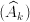and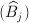, where now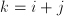denotes the cohort.
We can now predict the number of marriages per year, and per cohortHere, given the cohort, the shape of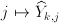is the following

```Yp=predict(reg,type="response")
tYp=matrix(Yp,nrow(m),ncol(m))
tYp[16,]
tYp[16,]
  10.00000 222.94525 209.32773 159.87855 115.06971  42.59102
  18.70168 148.92360```The errors (Pearson error) look like that
`Ep=residuals(reg,type="pearson")`(where the darker the blue, the smaller the residuals, and the darker the red, the higher the residuals). Obviously, we are missing something here, like a diagonal effect. But this is not the main problem here…I guess that study here is not valid. The problem is that we deal with open data, and numbers of marriages are not given here: what is given is a he proportion of marriage of men of ageduring the year, with a yearly normalization. There is a constraint on lines, i.e. we observe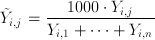so that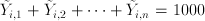This is mentioned in the titleIt is still possible to consider a Poisson regression on the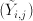, but unfortunately, I do not think any interpretation is valid (unless demography did not change last century). For instance, the following sum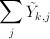looks like that

```apply(tYp,1,sum)
 919.948 838.762 846.301 816.552 943.559 930.280 857.871 896.113
 905.086 948.087 895.862 853.738 826.003 816.192 813.974 927.437```

i.e. if we look at the graphBut I do not think we can interpret that sum as the probability (if we divide by 1,000) that a man in that cohort gets married…. And more basically, I cannot do anything with that dataset…So open data might be interesting. The problem is that most of the time, the data are somehow normalized (or aggregated). And then, it becomes difficult to use them…

So I will have to work further to be able to write something (mathematically valid) on marriage strategy before Valentine’s day…. to be continued.